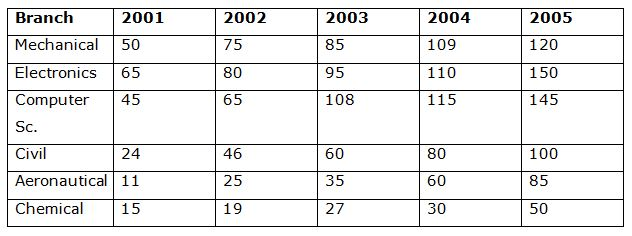# RBI Assistant Prelims Quantitative Aptitude Questions 2019-20 – (Day-03)

Dear Aspirants, Our IBPS Guide team is providing new series of Quantitative Aptitude Questions for RBI Assistant Prelims 2019-20 so the aspirants can practice it on a daily basis. These questions are framed by our skilled experts after understanding your needs thoroughly. Aspirants can practice these new series questions daily to familiarize with the exact exam pattern and make your preparation effective.

Ensure Your Ability Before of Exam

Take RBI Assistant Prelims Free Mock test

[WpProQuiz 7568]

DI

Direction (1 – 5): The following table shows the number of students in different branches from 2001 to 2005.1) What is the ratio of sum of students in Aeronautical and chemical to the sum of students in civil and electronics?

a) 35: 27

b) 119: 270

c) 191: 230

d) 357: 811

e) 47: 49

2) What is the average number of students in the year 2004?

a) 84

b) 86

c) 89

d) 90

e) 80

3) What is the percentage increase in students in 2003 as compared to previous year?

a) 23.56%

b) 27.98%

c) 37.88%

d) 26.89%

e) 32.26%

4) What is the difference between the total number of student in Electronics, Computer Sc. and Aeronautical together and the total number of student in Mechanical, Chemical and Civil together?

a) 205

b) 304

c) 403

d) 439

e) 216

5) In which year was the growth being maximum in Mechanical?

a) 2005

b) 2004

c) 2003

d) 2002

e) Either 2 or 4

Simplification

Direction (6 – 10): What value will come in place of question mark (?) in the following question?

6) 480/8- 12 * 5 + 3√343 = ?

a) 17

b) -3

c) 7

d) -9

e) 19

7) (65 /(616 x455))×77 x ? = 27

a) 1504

b) 1498

c) 1516

d) 1512

e) 1510

8) 60% of 110 + 88.88% of 243 + 55.55% of 99 = ?

a) 325

b) 316

c) 337

d) 313

e) 334

9) 71.42% of 154 + 28.57% of 77 = ?

a) 147

b) 132

c) 156

d) 111

e) 123

10) 80% of 635 – 15% of 700 = ?

a) 363

b) 403

c) 333

d) 383

e) 443

Directions (1-5):

Sum of Aeronautical and Chemical = 216 + 141 = 357

Sum of Civil and electronics = 500 + 310 = 810

Ratio = 357: 810 = 119: 270

Sum of students in 2004 = 504

Average = 504/6 = 84

Total number of students in 2002 = 310

Total number of students in 2003 = 410

Required percentage = [(410 – 310) / 310]* 100 = 32.26%

Sum of Students in Mechanical = 439

Sum of Students in Chemical = 141

Sum of Students in Civil = 310

Total Sum = 890

Sum of Students in Electronic = 500

Sum of Students in Computer Sc.= 478

Sum of Students in Aeronautical = 216

Total Sum = 1194

Required difference = 304

From the given figure:

Growth in mechanical students:

In 2002: [(75 – 50)/150] * 100 = 50%

In 2003: [(85 – 75)/175] * 100 = 13.33%

In 2004: [009 – 85)/85] * 100 = 28.23%

In 2005: [020 – 109)/109] * 100 = 10.09%

Directions (6-10):

? = (480/8)- 12 * 5 + 3√343

= 60 – 60 + 7 =7

? = (27 x 455 x 616)/(65 x 77)

= 27 x (455/65) x (616/77) = 27 x 7 x 8 = 1512

We note that 60% = 3/5, 88.88% = 8/9, 55.55% = 5/9

So, ? = 110 x (3/5) + 243 x (8/9) + 99 x (5/9)

= (5 x 22) x (3/5) + (9 x 27) x (8/9) + (9 x 11) x (5/9)

=(3 x 22)+(8x 27)+(5 x11)=337

We note that 71.42% = 5/7, 28.57% = 2/7

So, ? = 154 x (5/7) + 77 x (2/7)

= (7 x 22) x (5/7) + (7 x 11) x (2/7)

=(5 x22)+(2x 11)=132